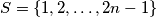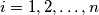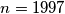### IMO Shortlist 1997 problem 4

Kvaliteta:
Avg: 0,0
Težina:
Avg: 0,0
An$n \times n$ matrix whose entries come from the set$S = \{1, 2, \ldots , 2n - 1\}$ is called a silver matrix if, for each$i = 1, 2, \ldots , n$, the$i$-th row and the$i$-th column together contain all elements of$S$. Show that:

(a) there is no silver matrix for$n = 1997$;

(b) silver matrices exist for infinitely many values of$n$.
Izvor: Međunarodna matematička olimpijada, shortlist 1997